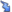# Help/TeX2pngPlugin

#### SynopsisHelp:WikiPlugin to display mathematical formulae in a Wiki page.

#### Usage

<?plugin TeX2png text="$$(a+b)^n=\sum_{k=0}^n{n\choose k}a^k b^{n-k}$$" ?>

gives

Cannot run TeX2png plugin, “/usr/bin/latex” does not exist

There is only one argument which is the text of the mathematical expression. This text must be enclosed by a dollar $within a paragraph or two dollars$$on a separate line. In the last case, all is centered. To write mathematical formulae, the syntax is the one ofLaTeX. #### Caveats This plugin is only to produce readable mathematical formulae. Any other text is not allowed : so if an expression is not enclosed by dollars then it will be displayed by a red text. It is all the same possible to display raw text as Cannot run TeX2png plugin, “/usr/bin/latex” does not exist by using : <?plugin TeX2png text="$\textrm{\LaTeX}\$" ?>

This plugin is not able to produce sophisticated mathematicals texts with links, cross references... For that, you can use for example LaTeX2html.

#### Examples

Some Greeks letters : Cannot run TeX2png plugin, “/usr/bin/latex” does not exist, Cannot run TeX2png plugin, “/usr/bin/latex” does not exist, ... and a formula Cannot run TeX2png plugin, “/usr/bin/latex” does not exist to test display in a paragraph.

Exercise 1 Consider the function Cannot run TeX2png plugin, “/usr/bin/latex” does not exist

1. Give the largest real domain for which f(x) is well defined.
2. Give a domain on which the function is one-to-one. Using this domain derive a formula for the inverse function Cannot run TeX2png plugin, “/usr/bin/latex” does not exist.
3. Calculate the derivative f'(x).

Exercise 2 Consider the function :

Cannot run TeX2png plugin, “/usr/bin/latex” does not exist
1. Show that for all r > 0 :Cannot run TeX2png plugin, “/usr/bin/latex” does not exist Help : you can use polar coordinates.
2. Hence find the limit of Cannot run TeX2png plugin, “/usr/bin/latex” does not exist as x tends Cannot run TeX2png plugin, “/usr/bin/latex” does not exist.